## 5.22Solving for energy

Examples with energy conservation in this chapter assume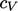is constant to produce the transport equation for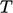in Eq. (2.65 ). Whencannot be assumed to be constant, it is common to solve an equation for internal energy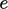instead, e.g. Eq. (2.60 ) with the material derivative replaced using Eq. (2.14 )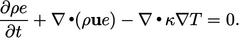(5.39)
This equation can be discretised to form a matrix equation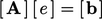, from which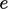can be solved. The challenge is that the diﬀusion term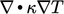is not expressed in terms of, so is discretised explicitly, which adversely aﬀects convergence.

To improve convergence, an implicit term is introduced which is similar in form and scale to the problem term. For energy Eq. (5.39 ), we use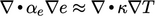, where the diﬀusivity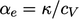is calculated from the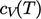function of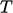.

The extra term is both “added and subtracted” in implicit and explicit form. This has the eﬀect of increasing the contributions to matrix coeﬃcients, while cancelling out a large part of the explicit contribution from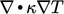, as illustrated below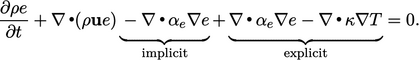The overall solution procedure involves ﬁrst updating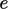from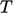from thermodynamic relationships, e.g. Eq. (2.62 ). The equation foris then solved, and the subsequent solution foris converted back to.

If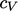is expressed as a polynomial function of, Eq. (2.64 ), thenis converted to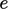using the analytical integral of Eq. (2.62 )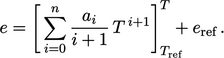(5.40)

### From temperature to energy

The conversion fromto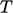is more complex becausecannot be made the subject of Eq. (5.40). Instead, it can be “inverted” using an iterative scheme such as the Newton-Raphson method,8 which for this problem is: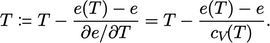(5.41)is updated from the currentusing the evaluated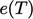and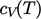polynomials, Eq. (5.40 ) and Eq. (2.64 ), respectively. One iteration is often suﬃcient for the error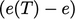to fall within a prescribed tolerance, but further iterations of Eq. (5.41) can be applied if necessary.

### Boundary conditions

The boundary conditions for the energy equation are generally speciﬁed in terms of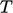. But since they must be applied to the variable being solved, they must be reposed in terms of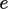. A ﬁxed value condition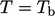is converted to an equivalent condition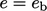, e.g. by Eq. (5.40 ). A ﬁxed gradient condition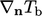foris converted to a ﬁxed gradient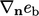forby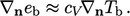(5.42)

8Isaac Newton,De analysi per aequationes numero terminorum inﬁnitas, 1669 and Joseph Raphson, Analysis aequationum universalis, 1690.

Notes on CFD: General Principles - 5.22 Solving for energy